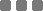## Thursday, August 20, 2015

### Math: Which One Is Bigger, 1000^1001 or 1001^1000?

The question looks like this:

10001001 (?) 10011000
We want to find the (?) operator.

I scribbled that a moment ago. I'm using logarithm, and captured the typed-steps as an image.

Let's start
Hang on for a moment...In conclusion

Without using calculator,
10001001 is larger than 10011000
`10001001 > 10011000`

Closure
As I observed, that pattern starts from integer greater than 2 (to any large positive integer).
I haven't tinkered the positive "infinity". I suppose it will be the same.
It's like imagination, the infinity concept.

And for number with, like, floating point, you can do your own scribbling.

Anyway, if we use 1 (in that pattern above):

12 (?) 21
1 (?) 2
1 < 2

If we use 2:
23 (?) 32
8 (?) 9
8 < 9

The next one, 3:
34 (?) 43
81 (?) 64
81 > 64

Next, 4:
45 (?) 54
1024 (?) 625
1024 > 625

And so on...

This technique will surely result different outcome for integer less than or equal to 2. Especially on the Let's assume it's 1 step (rounding down step).

Wolfram and Wikipedia